# Blaschke factor

(diff) ← Older revision | Latest revision (diff) | Newer revision → (diff)

Letbe the open unit disc in the complex plane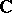. A holomorphic function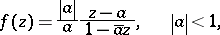onis called a Blaschke factor if it occurs in a Blaschke productThe defining properties of a Blaschke factor are:

a) a Blaschke factor has precisely one zero in;

b) a Blaschke factor has normon the boundary of.

The properties a)–b) may be used to define Blaschke factors on a Dirichlet domainin a Riemann surface as. Here,is the Green function forat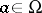andis its (multiple-valued) harmonic conjugate. See [a1] for the planar case.

Thus, in general a Blaschke factor will not be single valued, but it is single valued on simply-connected domains.

Next, for functions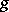of the Nevanlinna class (cf. Boundary properties of analytic functions), the term "Blaschke factor" is used to indicate the Blaschke product that has the same zeros as. For example, on the discone has the decomposition formula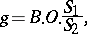whereis a Blaschke product or the Blaschke factor,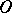is the outer factor, and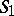,are singular inner functions; cf. [a2], Boundary properties of analytic functions; Hardy classes.

Similar decomposition theorems are known for domains in Riemann surfaces, cf. [a3].

How to Cite This Entry:
Blaschke factor. Encyclopedia of Mathematics. URL: http://encyclopediaofmath.org/index.php?title=Blaschke_factor&oldid=17194
This article was adapted from an original article by J. Wiegerinck (originator), which appeared in Encyclopedia of Mathematics - ISBN 1402006098. See original article